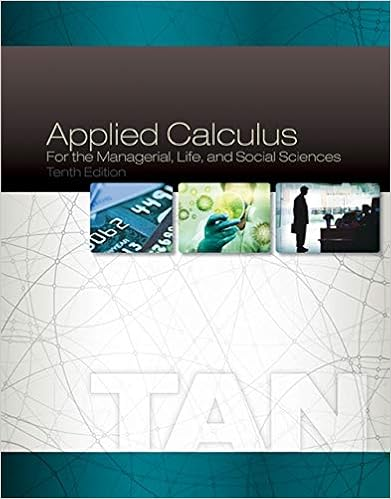# Test two review .PDF - MAT 1033 Test 2 Review Solve linear...

• Notes
• 4
• 25% (4) 1 out of 4 people found this document helpful

This preview shows page 1 - 3 out of 4 pages.

##### We have textbook solutions for you!
The document you are viewing contains questions related to this textbook.The document you are viewing contains questions related to this textbook.
Chapter 7 / Exercise 51
Applied Calculus for the Managerial, Life, and Social Sciences
TanExpert Verified
MAT 1033Test 2 ReviewSolve linear inequalities in one variable, including compound inequalitiesGraph linear inequalities in two variables.Solve a system of linear equations and inequalities in two variables by graphingSolve a system of linear equations in two variables using the addition method.Solve a system of linear equations in two variables using the substitution method.Solve applications involving systems of linear equationsSolve literal equations.Solve for the indicated variable.1)A=12bh for h2)P=2L+2W for L3)S=2rh+2r2for h4)V=13Bh for B15)H=75(a+2b); for b16)3x+4y=15 ; for y17)2x+7y=10x+1; for y18)L= (T - T0) +L0forTSolve , give your answer in interval notation
p
y
1
y
1
##### We have textbook solutions for you!
The document you are viewing contains questions related to this textbook.The document you are viewing contains questions related to this textbook.
Chapter 7 / Exercise 51
Applied Calculus for the Managerial, Life, and Social Sciences
TanExpert Verified
7
•••